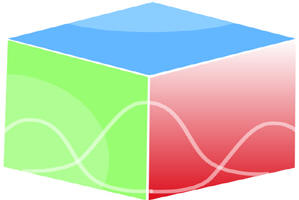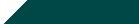``Home| Journals | Statistical Calculator | About Us | Contact UsUntitled Document

Fixed Point Theorem for Mapping Satisfying a Contractive Condition of Integral Type in D-Metric Spaces

Rajesh Suhag*

Research Article

Abstract: In this paper a fixed point theorem for mappings satisfying a contractive inequality of integral type in generalized metric space is established. This result is analogous to the result of Branciari.

Introduction: Dhage  introduced the notion of D-metric space (Generalized Metric Space) as follows.

A non-empty set X together with a function D : X ´ X ´ X ® R+, R+ denote the set of all non-negative real numbers, called a D-metric on X, becomes a D-metric space (X, D) if D satisfies the following properties :

(i) D(x, y, z) = 0 if and only if x = y = z (coincidence)

(ii) D(x, y, z) = D(x, z, y) = ….. (symmetry)

(iii) D(x, y, z) £ D(x, y, a) + D(x, a, z) + D(a, y, z) for all x, y, z, a Î X (tetrahedral inequality)

Example: Define a function D :: X ´ X ´ X ®R by D(x, y, z) = max {d(x, y), d(y, z), d(z, x)} for all x, y, z Î X and where d is an ordinary metric on X. Then D defines a D-metric on X.

A sequence {xn} in a D-metric space (X, D) is said to be D-convergent and converges to a point x Î X ifD(xm, xn, x) =0

A sequence {xn} in (X, D) is said to be D-Cauchy ifD(xm, xn, xp) = 0. A complete D-metric space X is one in which every D-Cauchy sequence converges to a point in it.

In a recent paper Branciari  established the following theorem.

Theorem : Let (X, d) be a complete metric space, C Î [0, 1), f : X®X a mapping such that, for each x, y ÎX,where f : R+ ® R+ is a Lebesgue-integrable mapping which is summable, non-negative, and such that, for each e > 0,dt > 0. Then f has a unique fixed point zÎX such that, for each xÎX,fnx = z.

The aim of this paper is to prove the above result of Branciari  in Generalized Metric Space.

Theorem: Let X be a complete D-metric space, k Î[0, 1), T : X®X a mapping such that, for each x, y, zÎX,…(1)

where f : R+ ® R+ is a Lebesgue – integrable mapping which is summable, non-negative and such that for each e > 0,> 0,  …(2)

Then T has a unique fixed point u ÎX such that, for each xÎX,= u.

Proof :- Let x Î X, and for brevity, define xn = Tnx

For each integer m ³ n ³ 1, from (1)£……£ kn…(3)

Taking the limit of (3), as n®¥, yields= 0   …(4)

which, from (2), implies that…(5)

We now show that {xn} is Cauchy. Suppose that it is not. Then there exists an e > 0 and subsequences {m(k)}, {n(k)}, {p(k)} such that

k £ m(k) < p(k) < n(k)

D(xm(k), xn(k), xp(k)) ³ e, D(xm(k), xn(k)-1, xp(k)-1)

< e       …(6)

Using the triangular inequality and (6),

D(xm(k)-1, xn(k)-1, xp(k)-1) £ D(xm(k)-1, xm(k), xp(k)-1)

+ D(xm(k)-1, xn(k)-1, xm(k)) + D(xm(k), xn(k)-1, xp(k)-1)

< D(xm(k)-1, xm(k), xp(k)-1)

+ D(xm(k)-1, xn(k)-1, xm(k)) + e    …(7)

Using (5) and (7)…(8)

Using (1), (6) and (8), it then follows that£ kwhich is a contradiction. Therefore {xn} is Cauchy, hence convergent. Call the limit u.

From (1)…(9)

Taking the limit of (9) as n®¥, we obtainwhich implies that= 0

which from (2), implies that D(Tu, u, u) = 0 or Tu = u. This implies u is a fixed point of T.

For uniqueness,

Suppose that u and v are fixed point of T. Then from (1),£ kwhich implies thatwhich, from (2), implies that D(u, u, v) = 0 or u = v, and the fixed point is unique.

Example: Let X = [0, 2] and D be a D-metric on X defined by D(x, y, z) = max{d(x, y), d(y, z), d(z, x)}, where d is a usual metric on X. Define T : X®X such that T(x) =. Let f : R+®R+ be such that f(t) = t, then all the conditions of theorem are satisfied and Pr Î[1/4, 1) and clearly 1 is the unique fixed point of T.

References:

               Dhage, B.C., Generalized metric spaces and mappings with fixed point, Bull. Cal. Math. Soc. 84, 329, 1992.

               Branciari, A., A fixed point theorem for mappings satisfying a general contractive condition of Integral type, Int. J. Math. Math. Sci. 29, no. 9, 531, 2002.